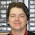## Monday, November 9, 2015

### HP Prime Geometry App Tutorial Part 7: Conic Sections and Equations

HP Prime Geometry App Tutorial Part 7: Conic Sections and Equations

In today’s lesson we will work conic sections and showing the equations for each of the conic sections.

Drawing an Ellipse and Display its Equation

Command:  ellipse(focus point 1, focus point 2, point on the ellipse)

Draw an ellipse with foci (1,4) and (5,4) and the point on the ellipse on (5,6).

1.  Press (Cmds), 5 for Curve, 5 for Ellipse.
2.  Go to the point (1,4) and press [ Enter ].
3.  Go to the point (5,4) and press [ Enter ].
4.  Go to the point (5,6) and press [ Enter ].

The next step is to display its equation.

5.  Press (Cmds), 8 for Cartesian, 5 for Equation of.
6.  Select the ellipse and press [ Enter ].  In this example the equation is named GD.
7.  To see the whole equation, press [ CAS ] and execute the equation(GD) command.
8.  Press the up button to highlight the equation and press (Show).  Scroll to see the entire equation.

Drawing a Hyperbola and Display its Equation

Command:  hyperbola(focus point 1, focus point 2, point on the hyperbola)

Drawn a hyperbola with foci (-2, -2) and (-4, 2) with point (-9, 2).

1.  Press (Cmds), 5 for Curve, 6 for Hyperbola.
2.  Go to the point (-2,-2) and press [ Enter ].
3.  Go to the point (-4,-2) and press [ Enter ].
4.  Go to the point (-9,2) and press [ Enter ].

The next step is to display its equation.

5.  Press (Cmds), 8 for Cartesian, 5 for Equation of.
6.  Select the ellipse and press [ Enter ].  In this example the equation is named GD.
7.  To see the whole equation, press [ CAS ] and execute the equation(GD) command.
8.  Press the up button to highlight the equation and press (Show).  Scroll to see the entire equation.

Drawing a Parabola and Display its Equation

Command:  parabola(focus point, directrix line)

Draw a parabola with focus point (0,-5) and (0,3) is on the directrix line.

1.  Press (Cmds), 5 for Curve, 7 for Parabola.
2.  Go to the point (0,-5) and press [ Enter ].
3.  Go to the point (0,3) and press [ Enter ].

The next step is to display its equation.

4.  Press (Cmds), 8 for Cartesian, 5 for Equation of.
5.  Select the ellipse and press [ Enter ].  In this example the equation is named GC.
6.  To see the whole equation, press [ CAS ] and execute the equation(GC) command.
7.  Press the up button to highlight the equation and press (Show).  Scroll to see the entire equation.

On the next part, we will work with rotating geometric objects.   Thank you and see you next time,

Eddie

This blog is property of Edward Shore – 2015.

1.I know it will sound stupid but in CAS mode, let's suppose in the setup I am set for the "Angle Measure" in DEGREES, it won't draw the ellipse. I will simply see the letter for the points A, B and C but no lines to draw the ellipse, nothing. I then go to settings (Shift + CAS/settings) and then change the angle measure for RADIANS, clear the screen and do again the Ellipse and NOW we see the ellipse to be drawn.

You may try it in the emulator on the PC, it does the same things. When set in RADIANS it draws the Ellipse, when in DEGREE it won't.

Both my Prime and the emulator are software version "2015 6 17 (8151)"

Strange isn't it. It does the same for you?

2.I did a little animated gif on this here: https://plus.google.com/+DenisBissonExplorerchess/posts/5QCkHm16oob

3.Also, it's annoying that they give the equation with a lowercase "x" and a lowercase "y".

Why? Because if we do a "COPY" of the resulting equation and then when to paste it in the app "Advanced Graphics", juste to validate and have the application plot the equation for us, we need to change manually all these x's and y's for capital ones since the app "Advanced Graphics" requires to have them in capital letters... It could have been nice if at both placed the accept the same either capital ones or lowercase ones... Don't you think?

### Retro Review: Radio Shack EC-2024

Retro Review:  Radio Shack EC-2024 Quick Facts Company:  Radio Shack Years in Production: Around 1994 Original Price: \$24.99 Bat...